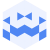Am 14. Mar. 2023 | 19:45 Uhr

# Mar 14 2023 | Tresor Linz | canceled but not canceled I Gischt I Polly MehrCreated at 30. Mar. 2023

70 Ansichten
##### by Tresor Linz

Tresor Linz - recorded on March 14 2023

<<<<<<<<<<<<<<<<<<<<<<<<<<<<<<<<<<<<<<<<<<<<<<<<<<<<<<<<<

Gischt
<<<<<<<<<<<<<<<<<<<<<<<<<<<<<<<<<<<<<<<<<<<<<<<<<<<<<<<<<

Ursula Winterauer aka Gischt is a composer and producer for electronic music based in Vienna. Within her artistic practice she generates raw sounds between brutality and atmospheric diffusion. Her eclectic soundscapes deliver differentiated interpretations of the genres industrial, techno and ambient, which are reflected by means of bass guitar, synthesiser and electro-smog clouds. Her works have been performed at various Austrian festivals like donaufestival, musikprotokoll, unsafe+sounds, as well as in in cities such as Zurich, Berlin, Priština, Riga and New York. Winterauer has collaborated with artists from various fields, including Annja Krautgasser, Claudia Larcher, Elisabeth B. Tambwé, Conny Zenk and Antoinette Zwirchmayr. In addition to curatorial work, she runs the Ventil Records label.

<<<<<<<<<<<<<<<<<<<<<<<<<<<<<<<<<<<<<<<<<<<<<<<<<<<<<<<<<
Polly Mehr
<<<<<<<<<<<<<<<<<<<<<<<<<<<<<<<<<<<<<<<<<<<<<<<<<<<<<<<<<
Polly Mehr ist das Solo Projekt der Künstlerin Julia Vogt (auch Sängerin und Texterin der Riot Grrrl Punk Band Musheen). Stimmlich zeigt sie sich von ihrer melodischeren Seite, begleitet von geloopten Lo-Fi Synthiklängen.

<<<<<<<<<<<<<<<<<<<<<<<<<<<<<<<<<<<<<<<<<<<<<<<<<<<<<<<<<
Tresor Linz
<<<<<<<<<<<<<<<<<<<<<<<<<<<<<<<<<<<<<<<<<<<<<<<<<<<<<<<<<

Raum für Klangkunst und künstlerische Experimente.

Space for sound art and artistic experiments.

##### Share & Embed
Embed this Video